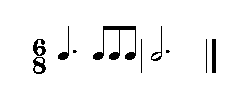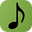The kinds of measures we have studied so far use what is known as simple meter. With simple meters, each beat is subdivided in equal halves. In the case of a 4/4 meter, each beat is divided into two eighth notes, as we saw earlier.

With compound meters, each beat is subdivided into three equal parts. We can tell a compound meter because it uses 6, 9 or 12 for the top number of the time signature. Let’s take a look at several examples of the compound meter of 6 over 8 (6/8). This meter actually has two beats; the note value that has the value of a beat is the dotted quarter note (crotchet), which can be subdivided into three eighth notes (quaver).Visit the Reference Section for more information.

Translated by Dan Román, revised by Sue Talley.